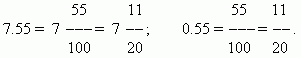# Converting a decimal to a vulgar fraction and back

To convert a decimal to a vulgar fraction it is necessary: a number after a decimal point to make as the numerator, and the n-th power of 10 ( here n – a quantity of decimal places ) - as the denominator. A non-zero integer part of a decimal is saved the same in a vulgar fraction; a zero integer part is omitted. For example:To convert a vulgar fraction to a decimal it is necessary to divide a numerator by a denominator according to the division rules.

E x a m p l e .   Convert  5 / 8  to a decimal fraction.

S o l u t i o n .   Dividing 5  by  8, we’ll receive 0.625.  ( Check it, please ! )

In the most of cases this process can be continued infinitely. Then a simple fraction cannot be converted exactly to a decimal. But in practice this is never required. Dividing is broken if decimal places, that are of interest, have been already received.

E x a m p l e . Convert  1 / 3  to a decimal fraction.

S o l u t i o n . Dividing 1 by 3 will be infinite: 1:3 = 0.3333… . Check it, please.